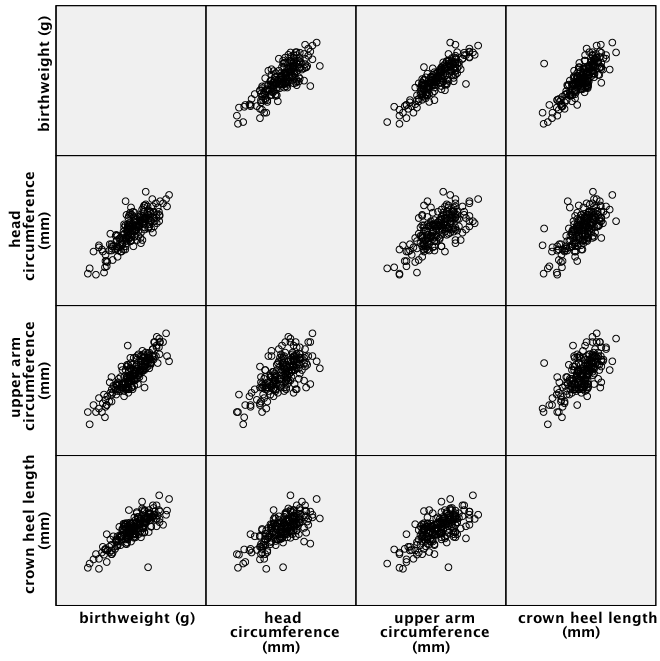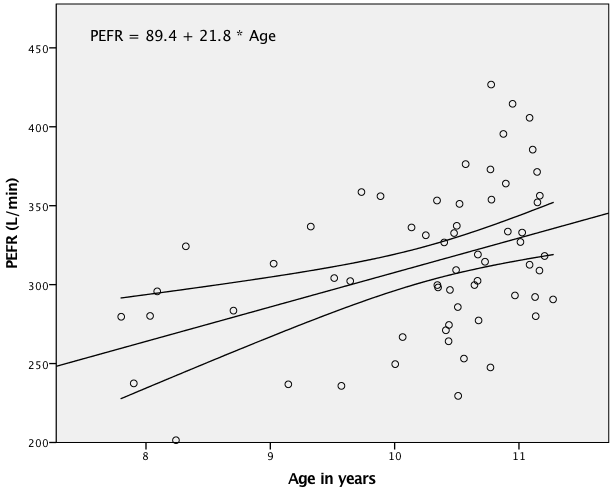﻿ Chapter 9: Analysing relationships between variables using SPSS

# Chapter 9: Analysing relationships between variables using SPSS

### Box 9.3 Presenting the results for Pearson’s correlation### Figure 9.3 Scatterplots for several variables### Box 9.5 Presenting correlations between several variables### Figure 9.5 Presenting scatterplots (a) with skewed data and (b) where data are transformed### Figure 9.6 Output for a rank test### Figure 9.7 Scatterplot of two variables with linear regression line### Figure 9.8 Output for simple regression### Box 9.11 Presenting the results for several predictor variables### Figure 9.11 Instruction

SPSS can not automatically produce this output.

### Box 9.13### Box 9.13 Instruction

SPSS can not automatically produce this output.

### Figure 9.9 Scatterplot of two variables with linear regression line and 95% confidence intervals